# Glass Calculation

Every type of glass has the properties necessary for its intended purpose, e.g. an optical glass has a particular refraction and an appliance glass has a particular chemical resistance. However, the properties of a glass, especially its viscosity, play a crucial part in production or moulding in particular.

These properties are continuously monitored in every operation, and a large quantity of equipment is required for this. On the basis of the glass composition, it is possible to calculate some of the properties of the glass.

The following explains in detail some of the parameters that will be calculated in the analysis:

## Viscosity

Viscosity is the most important property, since it is of the greatest significance for the manufacturing and processing of glass. Viscosity is a temperature-dependent parameter which is determined by the mobility of the structural particles of the substance in question. The forces to be overcome with this type of movement of particles are frictional forces. Viscosity is therefore also described as inner friction.

The movement of individual particles or components is made possible by the tearing apart of bonds. The energies necessary for this are applied in the melting process through thermal energies. If the temperature is increased all the time, the number of torn bonds increases and the viscosity is reduced. As the melt cools down, the bonds close again, which increases the viscosity.

The (dynamic) viscosity is designated using the symbol 'eta' and represents the force that is necessary to displace two parallel surfaces at a particular distance at a particular speed. If the viscosity is related to density, we talk about the kinematic viscosity. However, it is essential for glass production and glass processing to have corresponding reference or fixed points for the entire temperature and viscosity range applied during glass manufacture. It is impossible to manage without these.

These defined fixed points are derived from particular stages in the glass production and are used primarily for determining the viscosity values of the glass that are most favourable for these production stages.

In addition, there are empirically determined key figures which are used in practice, since these affect the setting of the production machinery. Since the viscosity of the glass changes by more than 13 decimal powers, the logarithm of the viscosity must be plotted as a function of the temperature.

If the viscosity curve runs steeply from 103 to 106 dPa s, the temperatures for the corresponding viscosities are relatively close to each other, i.e. the temperature range for processing the glass is short. Glass manufacturers refer to a glass of this type as being 'short', with the opposite type of glass being 'long'. The size of this temperature range is described as the length of the glass.

## Glass formation

When the batch is heated, the batch components go through a series of interconnected individual processes which are both technical/physical and chemical in nature which affect glass formation. These reactions can be divided up as follows:

• Solid body reactions in the area of the grain contacts (silicate formation, CO2 development)
• Formation of carbonatic melts which encase the quartz grains
• Decomposition reactions which create bubbles (CO2...)
• Dissolution reactions of the carbonatic melts with quartz grains (formation of silicate melts)

The temperature rises constantly. Under the temperature conditions of the batch melting, these reactions take place more or less at the same time. The formation silicate melts is completed for Na2O-CaO-SiO2 glasses at approx. .1000°C, for PbO-SiO2 glasses at approx. 800°C and for Na2O-B2O3-SiO2 glasses at approx. 1.100°C.

A further temperature increase in the melt is necessary, however, in order to achieve good homogenisation and refining of the glass in a reasonable time.

## Relative Machine Speed RMS

The RMS is used in the production of certain container glasses and compares glasses with the above glass as regards their production behaviour.

## Working Range Index

If the Working Range Index (WRI and Devitrification Index) >160, this characterises a low devitrification index; if it is <160, devitrification is more probable. The Devitrification Index was introduced for this reason: If D is positive, devitrification is improbable, if D is negative, devitrification is more likely.

## Gob Temperature

The Gob Temperature is the temperature at which the glass has a viscosity of approx. 1000 dPa s. At this temperature, an optimum gob forms. Most machine operators prefer values <1200 °C.

## Density

For the practical application of glass as a consumer object, the density is not particularly important. What is more interesting is the fact that the density is used as a calculation value for further properties and that density measurements can be used in practice to carry out, in a simple way, monitoring of the constancy of the glass composition.

Glasses with a high density are generally glasses with a high lead oxide content, which particularly includes optical glasses.

Because the density depends on volume, there are differences as regards the heating history of glasses. A tensioned glass (mainly tensile stress in this case) undergoes an expansion which causes an increase in volume. The density of a tensioned glass is thus approx. 0.02 less than that of a destressed glass.

Considerable significance is assigned to density in combination with temperature, since it has a direct effect on glass flows in glass melting furnaces and furnaces.

The density decreases as the temperature rises, which is due to the fact that the cohesion of the individual components of the glasses is considerable reduced, so that thermal vibrations start which means that the volume expands.

## Specific heat

Glass manufacture is a very heat-intensive process, which means that the thermal properties, especially specific heat, are very important.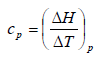The "true" specific heat at constant pressure is defined as a change in the enthalpy (heat retention) DH with the temperature DT

In practically all calculations, however, we are interested in what is called the "average" specific heat capacity tp_m (at constant pressure), which is determined between two temperatures T1 (mostly 0C or 20C) and T2:The "true" specific heat can also be determined from this equation

## Optical properties

For optical glasses, certain key figures are important for evaluation of the individual glass types as regards their use in optical systems and in their calculation.

If a ray of light from air in which it has almost the maximum speed of c_0 hits a glass, it reduces its speed to c as a result of the interplay of the light with the ions making up the glass. If the light falls vertically, the course of the light does not change; but if it falls at an angle, a deflection occurs which is covered by the law of refraction; here, alpha or beta represents the angles of the ray of light in relation to the normal in air or in the glass and n is the refraction index of the glass.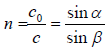The main refraction index given is the refraction index ne, or sometimes the refraction index nd.

The refraction index depends on the wavelength of the light, the temperature and the pressure. It is therefore usual to give the value for n for 20°C and 1013.25 mbar. The wavelength for which a refraction index applies is attached as a letter index to the n. In technical optics, 13 refraction indices are applied for all sorts of different wavelengths of the spectrum.

Dispersion of light is closely associated with the light refraction. In the language of technical optics, dispersion is understood to mean the difference in the refraction indices of a medium for light of various wavelengths. The difference n_f-n_c is described as the average dispersion d.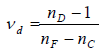The relative dispersion is described by the ratio (n_f-n_c)/(n_d-1). The reciprocal relative density is described as the Abbe coefficient ny_d.

Apart from the major significance that light refraction and dispersion have for optical glasses, these properties are also interesting for the commercial glass industry, especially for lead glasses. High refraction values increase the shine of these glasses, which finally improves quality effectively.

For practical production, regular measurements of the refraction value can provide a relatively simple, sufficiently precise check on the consistency of the glass composition, since even minor deviations will lead to changes in the refractive value.

## Surface tension

The surface tension of glass is important during the glass melt and in several stages of manufacture. A particular within a body is attracted by all the neighbouring particles so that the resultant force is zero. If, on the other hand, a particle is on the surface, then there are no forces of attraction on one side and a force in the direction of the interior is created.

In order to take a particle from the inside to the surface, a certain degree of work is required. Bodies with a large surface therefore have more energy and are attempting to take on a state of less energy by reducing their surface area. Liquids therefore take on a spherical shape if possible, since the ratio of surface to volume is lowest in this form.

The enlargement of a surface assumes that particles will be transported from the inside to the surface, which requires an expenditure of energy. The energy that is required to form a new surface is described as specific free surface energy. Generally, the designation surface tension sigma is commoner.

## Thermal expansion

The thermal expansion of glass is of major practical significance, especially as regards the lasting, break-proof melting of various glasses with each other and with other substances. A precise knowledge of the progress of the thermal expansion of glasses is therefore an essential precondition for controlling some of the technological processes in glass production and processing.

The increase in volume of a body through thermal expansion happens on all sides. All isotropic bodies, including glass, expand at the same time in all directions to the same extent. This thermal expansion of the glass is caused by the fact that, as the temperature increases, the thermal energy present in the glass increases and, consequently, the particles begin to vibrate more. Atoms linked with each other by forces thus spread further apart, causing expansion.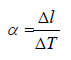Thermal expansion is designated by the average longitudinal expansion coefficient (coefficient of linear expansion) alpha . It is the fraction of the length by which a bar of the material in question increases in length following a temperature increase of 1 degree:

For the commonest substances, within the temperature limits that are technically interesting, the a value does not change. This means that the change in length is proportion to the change in temperature. However, the change in length in the case of glass is not exactly proportional to temperature, and the coefficient of thermal expansion is therefore temperature-dependent. In practice, the average coefficient of volume expansion (cubic expansion coefficient) beta is now used.

## Thermal conductivity

The thermal conductivity of glass is very low in comparison with other materials such as copper, aluminium and iron. It is therefore only important for particular areas of application.

Heat can be transported in glass through thermal conductivity and thermal radiation. At low temperatures the first process predominates, with the second occurring more at higher temperatures.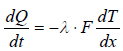According to the following definition equation, the thermal conductivity lambda is the quantity of heat dQ, which flows in the time dt vertically through the surface F at a temperature there of dT/dx.

In measurements, we generally obtain a total effective thermal conductivity which is made up of the figures for pure thermal conductivity and thermal radiation. Often, eff l is described as thermal conductivity for greater simplicity.

Because of the poor thermal conductivity of glass, certain products such as glass fibre matting, fibreglass and foam glass are used as insulation materials for all sorts of different applications. The thermal conductivity of foam glass is only approx. 1/20 of that of normal glass.

## Elastic properties

A solid body suffers deformation as a result of a deforming force. If this deformation goes back completely after the force is removed, the body is described as being ideally elastic. Hooke's law states that the deformation D is proportional to the stress S applied.

The proportionality constant M occurring in this equation is generally described as a modulus. Depending on the nature of the deformation, there are various moduli. Their values depend on the composition; the moduli therefore represent material constants.

Tensile stress creates an expansion which is designated as the modulus of expansion (or the modulus of elasticity) E. A shearing stress causes shearing strain. The corresponding modulus G has a number of designations: the modulus of rigidity, shear modulus, twist modulus, torsion modulus. Finally, pressure on all sides causes compression, with the modulus of compression K.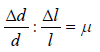With expansion, a transverse contraction occurs in the direction at right angles to this. If the relative expansion is dl/l and the relative transverse contraction is dd/d, then the ratio is described as the reciprocal of Poisson ratio

It can be deduced that the reciprocal of Poisson ratio my must lie between 0 and 0.5, A low reciprocal of Poisson ratio means that with a particular longitudinal expansion there is only a small transverse contraction.

## Electrical properties

The use of glass in electrical engineering has increased considerably in recent years. Here, it is particularly the electrical properties at normal temperatures that are important, whilst for electrical glass melting the properties at higher temperatures are important.

Glass is amongst the poorer electrical conductors. Whilst, with good electrical conductors such as metals, the current is transported via free electrons, conduction is via ions in the case of glass.

The capacity of a substance to allow the transport of current by freely moving electrons or ions is described as its electrical conductivity. The electrical conductivity of a material is dependent on its specific electrical resistance rho.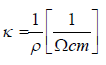The specific electrical conductivity kappa (or sigma) of a material is designated as the reciprocal value of the specific electrical resistance.

As a consequence of its high rho value, glass is used as an electrical insulating material. As the temperature increases, the rho decreases, which is due to the fact that the conductive ions, especially the Na+ ions, become more mobile, thus improving conductivity.

Because kappa, due to the ion conductivity of glass, is also extremely temperaturedependent, every glass has very different values. At room temperature, a glass of this type, for example, has a kappa value of 10-13 to 10-14 Ohm-1 cm-1. In the melt temperature range, kappa increases to 0.1 to 0.3 Ohm-1 cm-1. In practical use, the t_R8 value is often used for operating check measurements. This value is the temperature at which kappa=100 10-10 Ohm-1 cm-1.

This temperature varies, depending on glass composition, which means that if there is a change in the measured value, there are likely to be faults in the composition of the glass. Both parameters, rho and kappa, are material constants which have characteristic values for glasses of different compositions.

## Dielectric properties / permittivity

If a dielectric is placed between the plates of a capacitor, its capacity C increases compared to the capacity V C measured in a vacuum to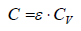In this equation, the proportionality factor epsilon is described as the permittivity coefficient epsilon_r or epsilon. The permittivity coefficient is better known as the dielectricity constant or figure.

The cause of this effect is that displacements of charges occur under the effect of an electric field. With an ion, the electron sheath can be deformed, or whole ions can be displaced in their location or can take up completely different positions. The first possibility is all the more likely, the greater the polarisability of the ion in question is. This means that there is a correlation with the refraction index for which a corresponding effect is decisive. For very high frequencies, the Maxwellian ratio applies with the refraction index n: e =n2 which is, however, not quite met for glass.

If a glass is inserted between the plates of a capacitor, not only is the capacity increased, but there is also a displacement of the phase angle between current and voltage. As the current passes through, the glass uses some electrical energy . the dielectrical losses. They become apparent in that the phase angle around the small angle theta becomes smaller than 90°C.

The quantitative dimension for this energy consumption is the tangent of this angle tan(theta), which is also described as the loss factor and which is equal to the proportion of effective power to idle power.

## Strength

The tensile strength is one of the most important of the mechanical properties of glass. It has a major effect on the limit of usability of the glass as regards all sorts of mechanical stresses and also determines the temperature change resistance (TCR) to a large extent.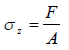The tensile strength is determined by the force F which tears a glass rod with the cross-section A.

A F z s = The tensile strength is determined by the force F which tears a glass rod with the cross-section A.

In comparison to other materials such as steel or cast steel, glass has a low tensile strength. The tensile strength of glass calculated theoretically from the bonding forces gives a value that is almost a hundred times the practical measurement results. This is due mainly to the structural micro-cracks and loose areas which also cover the glass surface as notchshaped unevennesses.

The high risk of fracture of glass following blows and impacts is due to the insufficient tensile strength. If a glass surface is exposed to blows and impacts, it suffers indentations which lead to an enlargement and expansion of the surface, causing tensile stress. With most glasses, even light impacts are enough to exceed the elasticity limit, which leads to an immediate fracture.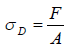The tensile strength is determined by the force F which tears a glass rod with the cross-section A.

As a result of the relatively good D s values, glass can be used as a building material in the form of glass bricks and similar.

Glass is relatively insensitive to compressive stress, and this property is used in practice by glass having high compressive stresses which considerably improves the tensile strength. These stresses are created by rapid cooling of the surfaces, especially of flat glasses, producing safety glass and prestressed glass products.

With these special glass products, these technological treatments considerably increase impact resistance.